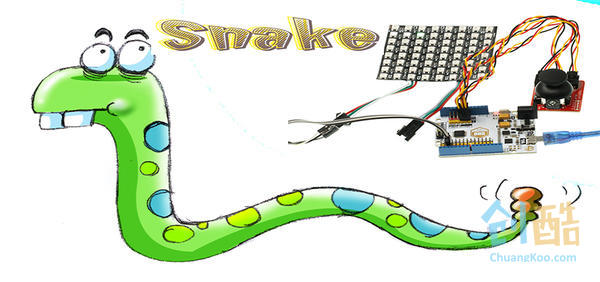### 8*8 Rainbow LED Matrix Snake Game

LUCY 发布于 2018-01-20 12:16Remember the snake game we played on our mobile or game box during our childhood? Today we are going to learn how to use Flexible Rainbow 64 RGB 8*8 LED Matrix to make a snake game. We use Joystick breakout module to control it.

3 X Jumper Cable

Arduino IDE

Connect Flexible 64 RGB 8*8 Rainbow LED Matrix to portThe code we are going to make includes the following sections:

1. Generate food;
2. Time Delay;
4. Gameover, display the score;
5. Display start-up picture;
6. Snake Movement;
7. Calculate the score. Every 5 food eaten will increase snake speed for one time. But we have to limit the maximum speed.

We use Random Number Function to produce two random numbers for X axle and Y axleof the food separately. We have to judge whether the axleof food and the axleof snake are the same. If it is the same, we need to regenerate food.

Code Example:

[cceN_cpp theme="dawn"]
A:

FX = random(0, 7);

FY = random(0, 7);

for (int i = 0; i < 3 + socre; i++)

{

if ( FX == sx[i] && FY == sy[i])

goto A;

}
[/cceN_cpp]

Set A0-A2 to be input mode and read its voltage. The middle value is around 510. We have to judge the value and assign it with relative movement.

Code Example:

[cceN_cpp theme="dawn"]

if (yValue > 950 && KEY != 5) {

K = 8;

}
[/cceN_cpp]

Firstly, we have to judge which direction the snake moves. Snake move is the axledata shift. That means the second section axleof snake equals the axleof snake head and the third equals the second. According to this rule, we assign the snake head new value. We have to pay attention that the snake body surpassed the screen frame will appear in the opposite frame.

Code Example:

[cceN_cpp theme="dawn"]
if (KEY == 8) // Snake upward

{

for (int i = 2 + socre; i > 0; i--)

{

sx[i] = sx[i - 1];

sy[i] = sy[i - 1];

}

sy = sy - 1;

if (sy < 0) // The part of snake body surpassed screen frame will appear in the opposite frame.

sy = 7;

}
[/cceN_cpp]

We only have to judge whether the axleof snake head equals the axleof food. In other words, the food was eaten.Then we have to generate food axleand display it. At the same time, we have to judge whether the score of the game is a multiple of 5. If it is, then increase speed for one time and decrease delay time.

Code Example:

[cceN_cpp theme="dawn"]
if (SY == FY && SX == FX)

{

RANDOM();

socre ++;

Colour = 40; // Colour = 0;

Colour = 0;

c = strip.Color(Colour, Colour, Colour);

x = light[FX][FY]; //Display Food

strip.setPixelColor(x, c);

strip.show();//Send data

if ( !(socre % 5)) { //Increase snake speed according to the score. Every 5 food eaten, increase snake speed by 100ms.

speedS = speedS - 50;

if (speedS < 150) // The minimum speed is 200ms. If snake speed lower than 200ms, the speed remains unchanged. speedS = 150;

}

}
[/cceN_cpp]

When snake head touches snake body, game is over. And we get the score by judging whether the axle of snake head equals to the axle of snake body.

Code Example:

[cceN_cpp theme="dawn"]
for (int i = 1; i <= 2 + socre; i++)

{

if ( SX == sx[i] && SY == sy[i])

gameover();

}
[/cceN_cpp]

## 作者的最新作品